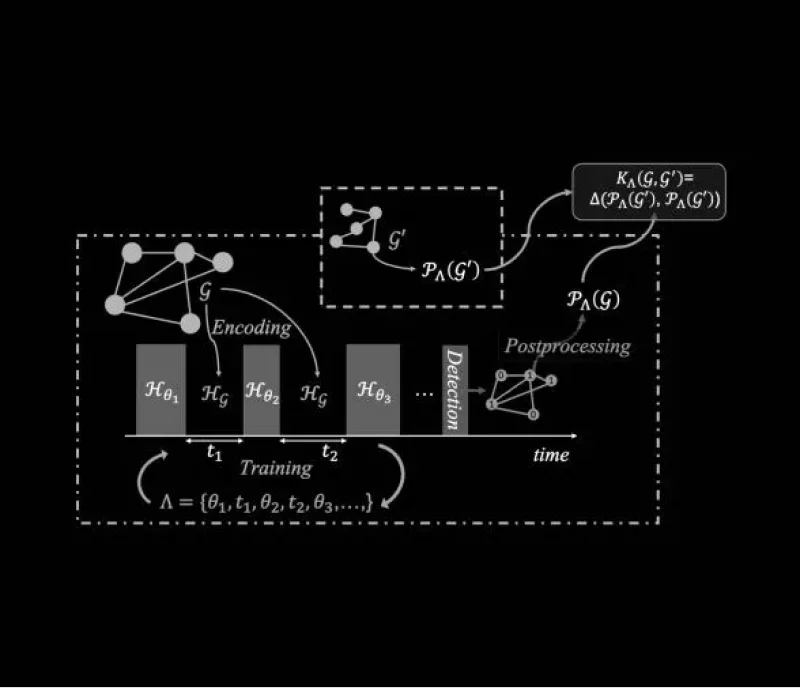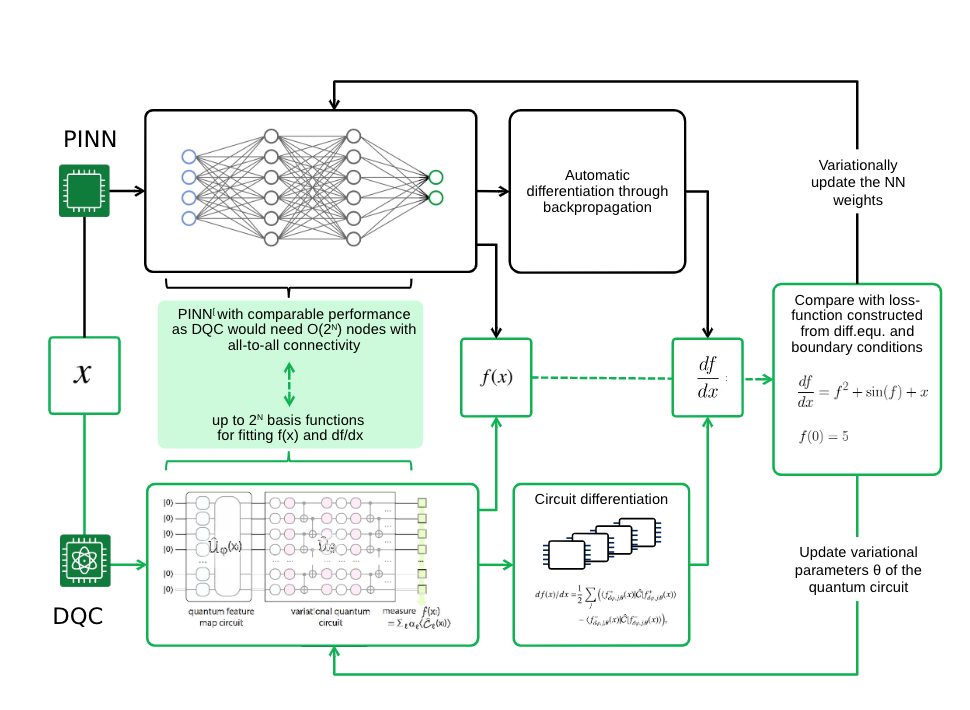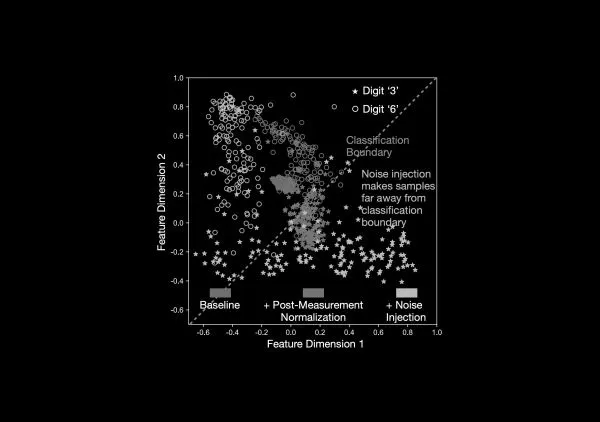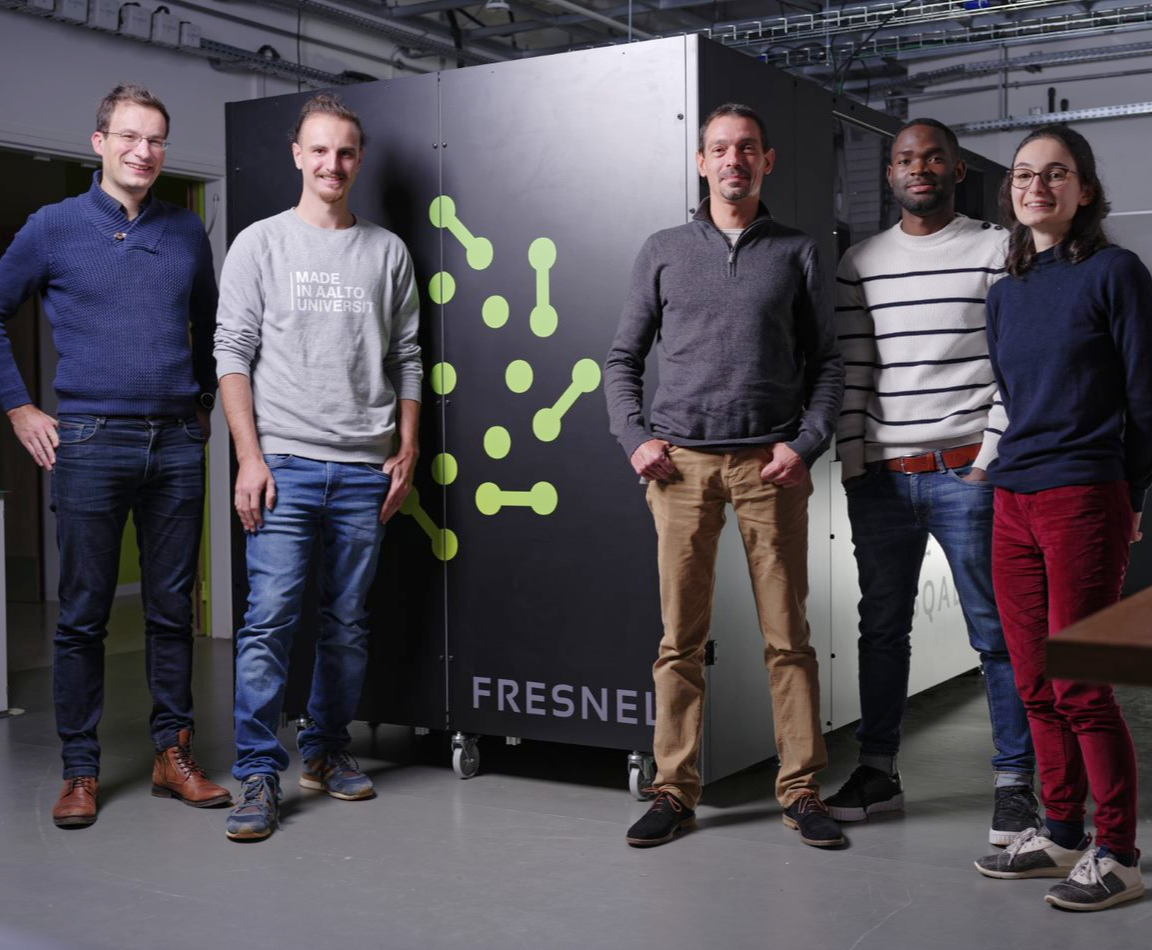# Algorithms

## Quantum Evolution Kernel

In many fields, such as chemistry, bioinformatics, social network analysis and computer vision, key information is encoded in terms of graphs. Thus, a large body of work in classical machine learning has been devoted to studying relationships between graphs, also known as graph kernels. The graph kernel approach is based on finding a way to associate any graph with a feature vector encapsulating its relevant characteristics (the feature map) and then computing the similarity between those vectors, e.g., via scalar products in the feature space. Despite some interesting results in recent years, the algorithmic complexity of the most valuable kernels makes them difficult to apply in the case of large graphs.

A versatile and easily scalable graph kernel method known as the Quantum Evolution Kernel (QEK) was introduced by PASQAL in 2021. The central idea of this method is to encode information about the graph in terms of the parameters of a Hamiltonian acting on an array of qubits, and defining the kernel as overlap between output states (or their properties) produced by two different graph cases; with quantum computer’s ability to efficiently produce complex output states and compute wavefunction overlaps naturally, this poses a potential advantage over known classical methods.

While QEK was specifically applied to classification and regression tasks, it could also be used for clustering and as subroutine in other algorithms. Furthermore, there are many parallels to Graph Neural Networks due to the kernel-neural network correspondence, opening up the potential to apply QEK-like algorithm paradigms also to more general graph learning problems.## Differentiable Quantum Circuits

Differential equations (DEs) are mathematical models describing how quantities in physical reality interdepend on each other and how they evolve over time. Understanding such relationships is critical for solving important problems across virtually all engineering and scientific disciplines, with applications ranging from fluid dynamics to epidemiology. Exact solutions are rare, and solving these equations often involves numerical techniques consuming enormous computational resources. In fact, solving for systems presenting non-linearities, many variables, or instabilities is often beyond the present-day capabilities of classical computers.

PASQAL proposes a novel variational method for solving DEs: the differentiable quantum circuits (DQC) strategy. Since its original inception, multiple derivative works have been added to the portfolio, including learning differential models from data (QMoD), solving stochastic differential equations (QQM/DQGM), efficiently training generative models (DQGM), integral transformations (AutoInt) and design optimization (QEL) of systems governed by differential equations. Together, these constitute a family of techniques we coin Quantum Scientific Machine Learning, extending the rapidly growing field of classical SciML.

## Scientific resources

Breakthroughs and research papers

Our teams publish on a regular basis content related to scientific findings and concrete Quantum Computing applications.## Interested in World-Class Research?

Read on some of our team’s world-class research on quantum solutions